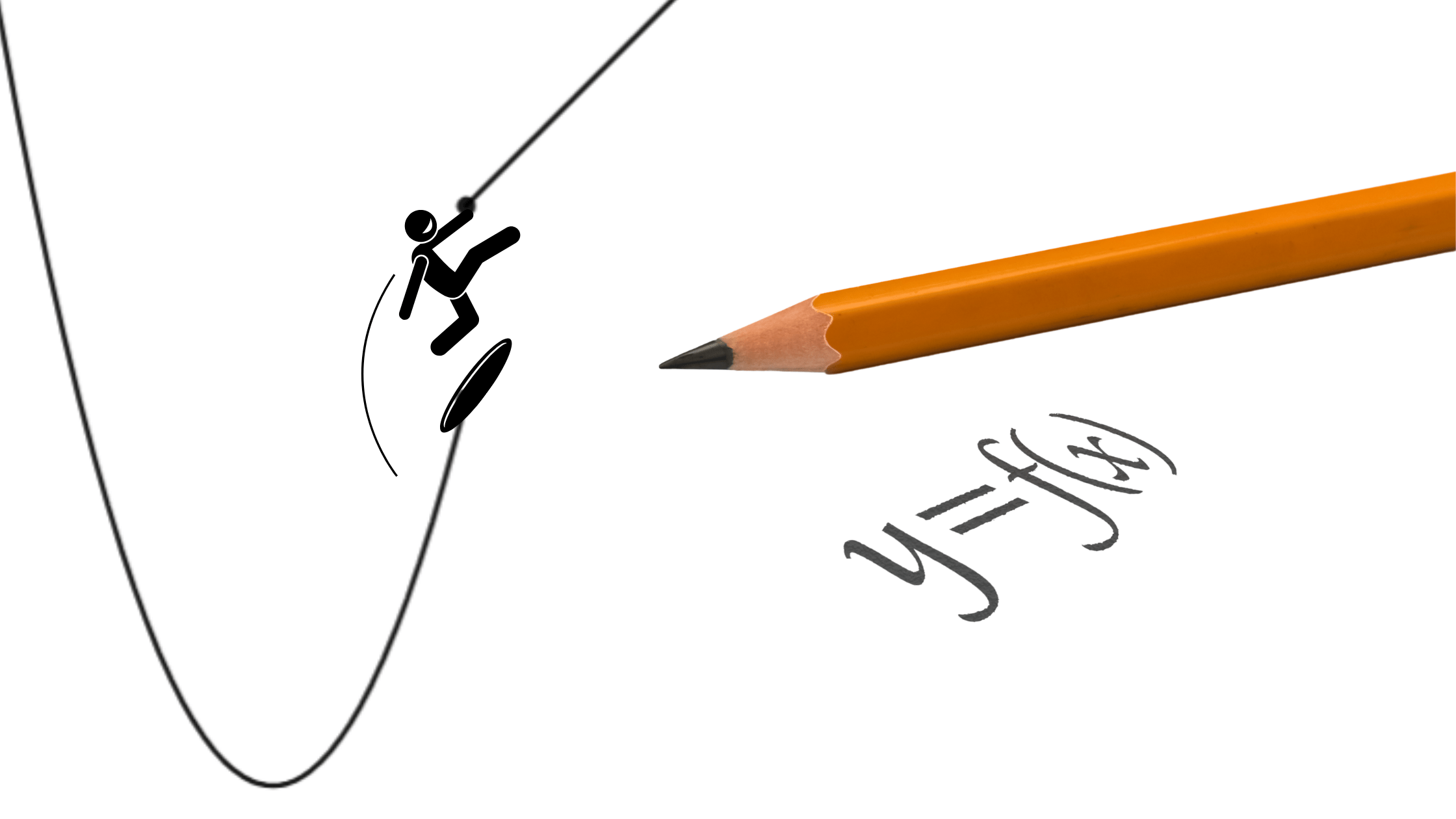# Calculus: Discontinuity and LimitsA function can be continuous or discontinuous. There are different types of discontinuities that we will go over here. We will also show you how to determine a limit of the function based on each type of discontinuity.

A function is continuous when the function is defined at every point and when a two-sided limit can be determined for every input.

For example, the function is a continuous function.

If we wanted to determine the limit as x approaches any value within this function, every such limit would exist.

From the graph we can see that  .

If we wanted to evaluate the limit algebraically, we would substitute the value of the input the function approaches into the function expression and evaluate.

## Point Discontinuity

Point Discontinuity occurs when a function is undefined as a single point. That point is called a hole.

A function will be undefined at that point, but the two sided limit will exist if the function approaches the output of the point from the left and from the right.

An example of a function with such type of discontinuity is a rational function where one factor can be completely eliminated (thus creating a hole):

The function above is discontinuous.

In order to determine the limit of the above function as the input approaches the value of 5, the expression of the function needs to be simplified first.

The two sided limit exists, however, the function is undefined at , since

## Removable Point Discontinuity

A Removable Point Discontinuity occurs when a function has a point discontinuity, but is defined at another, removed point for the same input.

For example, if we take the function equation from the previous example and turn it into a piecewise function that includes the value of the output for the same input as the discontinuity where the function is defined, it would look like this:

The limit of the above function as would still be the same (as determined in the previous example), as function pieces are still approaching the output of    from the left and from the right.

However, if we evaluated the function at now, because there is a removed defined output, the function is now defined at and .

## Jump Discontinuity

A Jump Discontinuity occurs when the function consists of pieces whose ends do not result in the same outputs for the same input. In order to ensure the relation is a function, the function would only be defined for one of the outputs for the same input.

For example, let’s consider the following piecewise function

Based on the equation of the above function, the function is defined at since for one of the pieces of the function the domain includes .

In order to determine we would substitute the value of 2 for into the linear piece of the function since this is the piece that contains a point where the function is defined at .

The two-sided limit as for this function does not exist (DNE), because the two pieces of the function approach two different outputs for the same input.

, so the

## Infinite Discontinuity

An Infinite Discontinuity occurs when there is a vertical asymptote and the function approaches that asymptote.

For example, a function  has a vertical asymptote at and, therefore, there exists an infinite discontinuity.

The function above is undefined at for every value of on both sides of , approaches positive infinity .

Even though the function is not defined at , the two-sided limit still exists, since the function approaches from the left and from the right.

Let’s also consider the following function with the Infinite Discontinuity.

There is a vertical asymptote at , however the two pieces of the function approach two different values from the left and from the right

Therefore, the two-sided limit will not exist